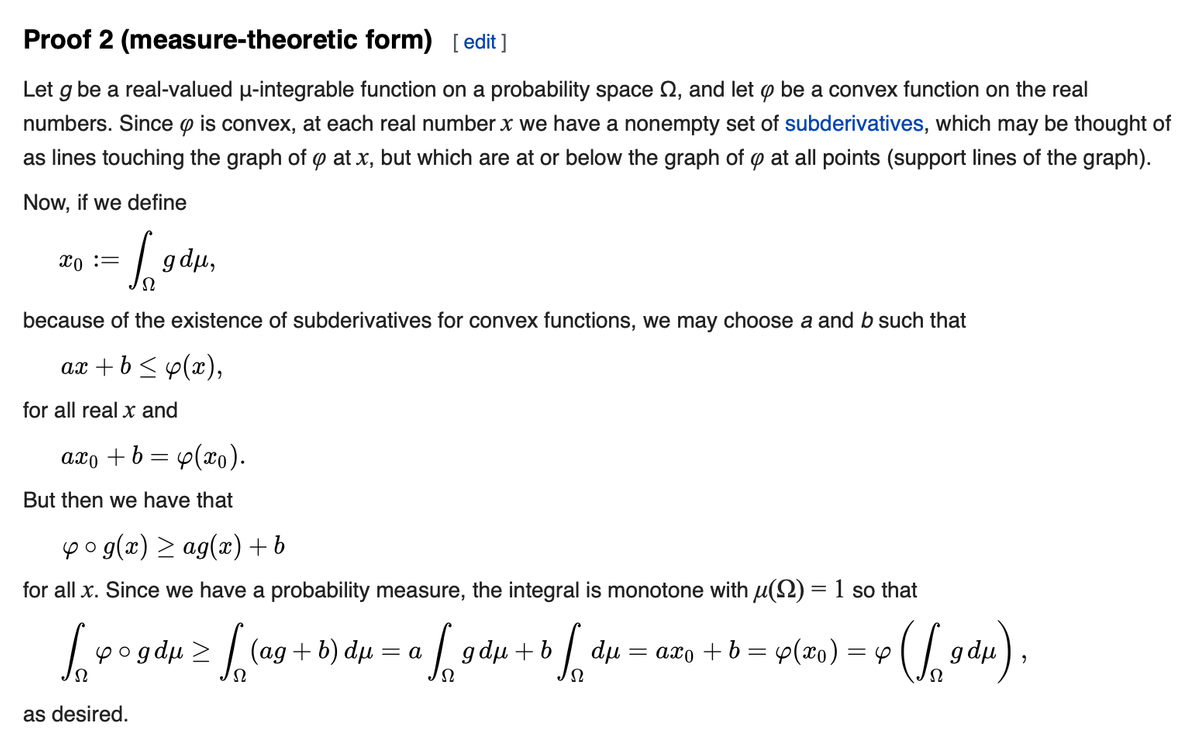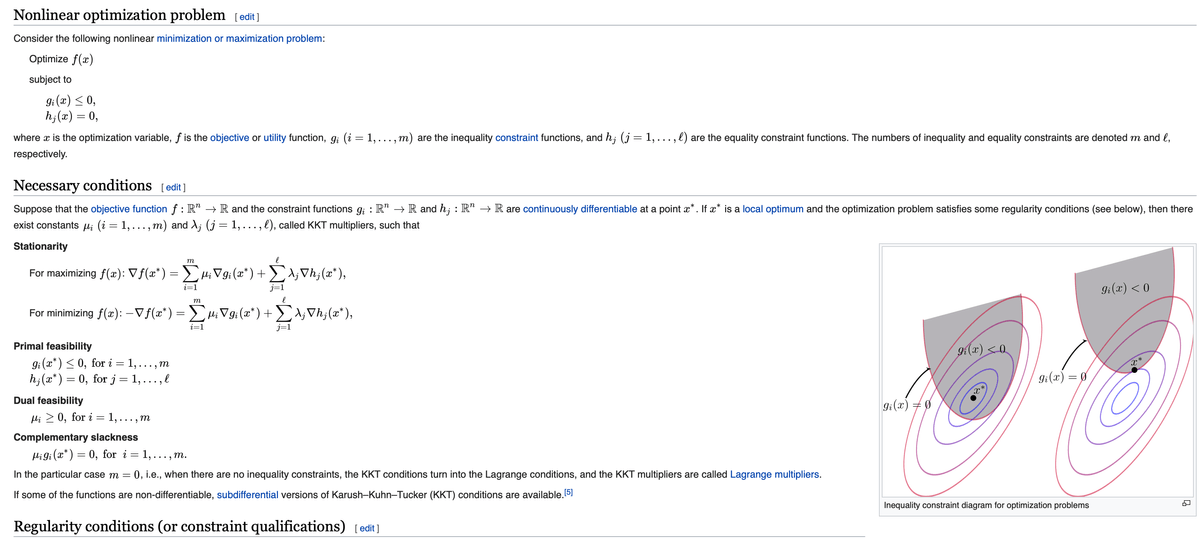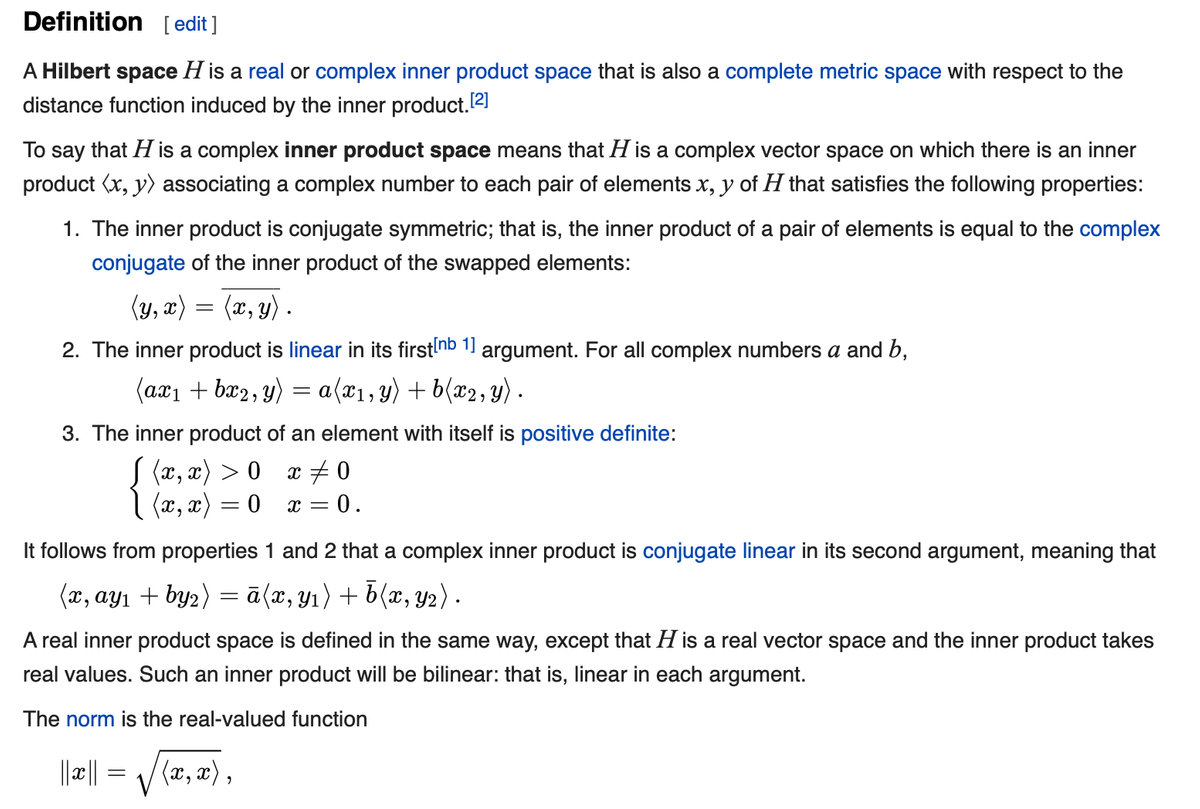# 《统计学习方法》 Note -Part1

## 读李航的《统计学习方法》（第2版)的收获

· 机器学习,阅读,数学

Jensen Inequality

In mathematics, Jensen's inequality, named after the Danish mathematician Johan Jensen, relates the value of a convex function of an integral to the integral of the convex function. It was proven by Jensen in 1906. Given its generality, the inequality appears in many forms depending on the context, some of which are presented below. In its simplest form the inequality states that the convex transformation of a mean is less than or equal to the mean applied after convex transformation; it is a simple corollary that the opposite is true of concave transformations.

https://en.wikipedia.org/wiki/Jensen%27s_inequality#Proof_1_(finite_form)- KKT condition

Karush-Kuhn-Tucker conditions

In mathematical optimization, KKT conditions are first derivative tests (sometimes called first-order) necessary conditions for a solution in nonlinear programming to be optimal, provided that some regularity conditions are satisfied. Allowing inequality constraints, the KKT approach to nonlinear programming generalizes the method of Lagrange multipliers, which allows only equality except in the few special cases where a closed-form solution can be derived analytically. In general, many optimization algorithms can be interpreted as methods for numerically solving the KKT system of equations and inequalities.

KKT, originally named after Kuhn and Tucker, who first published the conditions in 1951. Later scholars discovered that the necessary conditions for this problem had been stated by Karush in his master's thesis in 1939.- Gram Matrix

https://en.wikipedia.org/wiki/Gramian_matrix

In linear algebra, the Gram matrix (Gramian matrix or Gramian) of a set of vectors v1, ..., vn in an inner product space is the Hermitian matrix of inner products, whose entries are given by :

G_ij = <vi, vj>

An important application is to compute linear independence: a set of vectors are linearly independent if and only if the Gram determinant (the determinant of the Gram matrix) is non-zero.

- Hermitian Matrix

https://en.wikipedia.org/wiki/Hermitian_matrix

(埃尔米特矩阵、厄米特矩阵）

In mathematics, a Hermitian matrix(or self-adjoint matrix) is a complex square matrix that is equal to its own conjugate transpose -- that is, the element in the i-th row and j-th column is equal to the complex conjugate of the element in the j-th row and i-th column, for all indices i and j :

A Hermitian <==> a_ij = conjugate of (a_ji )

- Hilbert Space

https://en.wikipedia.org/wiki/Hilbert_space

The mathematical concept of a Hilbert space, named after David Hilbert, generalizes the notion of Euclidean space. It extends the methods of vector algebra and calculus from the two-dimensional Euclidean plane and three-dimensional space to spaces with any finite or infinite number of dimensions.

A Hilbert space is an abstract vector space possessing the structure of an inner product that allows length and angle to be measured. Furthermore, Hilbert spaces are complete: there are enough limits in the spaces to allow the techniques of calculus to be used.

Euclidean space: think about three dimensional vectors x and y.

x = (x1, x2, x3)' y = (y1, y2, y3)' ,

x · y = x1y1 + x2y2 + x3y3 ( dot product, inner product).

The dot product satisfies some properties:

* symmetric

* linear

* positive definite ( x · x >= 0, with equality if and only if x = 0 )

Definition:

A Hilbert space H is a real or complex inner product space that is also a complete metric space with respect to the distance function induced by the inner product.×